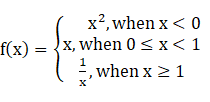IfFind:

(i) f($$\dfrac{1}{2}$$)

(ii) f(-2)

(iii) f(1)

(iv) $$f (\sqrt{3})$$

(v) $$f (\sqrt{-3})$$

Asked by Sakshi | 1 year ago |  99

##### Solution :-

(i) f ($$\dfrac{1}{2}$$)

When, 0 ≤ x ≤ 1, f(x) = x

f ($$\dfrac{1}{2}$$) = $$\dfrac{1}{2}$$

(ii) f (-2)

When, x < 0, f(x) = x2

f (–2) = (–2)2

= 4

f (–2) = 4

(iii) f (1)

When, x ≥ 1, f (x) = $$\dfrac{1}{x}$$

f (1) = $$\dfrac{1}{1}$$

f(1) = 1

(iv) $$f(\sqrt{3})$$

We have $$\sqrt{3}$$ = 1.732 > 1

When, x ≥ 1, f (x) = $$\dfrac{1}{x}$$

$$f( \sqrt{3})$$$$\dfrac{1}{ \sqrt{3}}$$

(v) $$f(- \sqrt{3})$$

We know $$- \sqrt{3}$$ is not a real number and the function f(x) is defined only when x ∈ R.

$$f(- \sqrt{3})$$ does not exist.

Answered by Sakshi | 1 year ago

### Related Questions

#### Let R = {(a, b) : a, b, ϵ N and a < b}.Show that R is a binary relation on N

Let R = {(a, b) : a, b, ϵ N and a < b}.Show that R is a binary relation on N, which is neither reflexive nor symmetric. Show that R is transitive.

#### Let A = (1, 2, 3} and B = {4} How many relations can be defined from A to B.

Let A = (1, 2, 3} and B = {4} How many relations can be defined from A to B.

#### Let R = {(x, x2) : x is a prime number less than 10}.

Let R = {(x, x2) : x is a prime number less than 10}.

(i) Write R in roster form.

(ii) Find dom (R) and range (R).